# E-PolyLearning

 16. Following number is called the __________ number.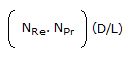a. Peclet b. Stanton c. Graetz d. none of these

 17. What is the logarithmic mean of r1 and r2 ?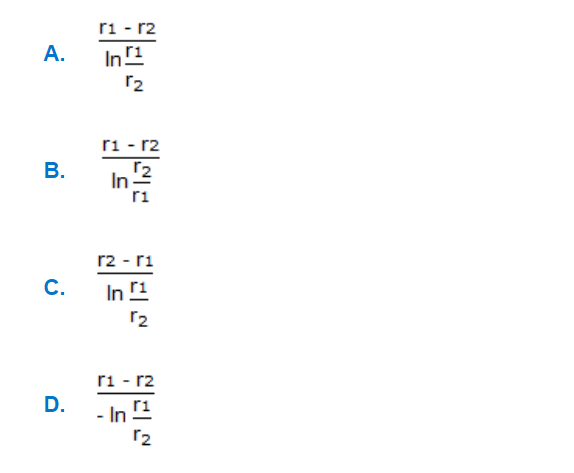a. A b. B c. C d. D
 18. The following equation is the __________ analogy.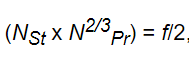a. Colburn b. Reynolds c. Prandtl d. None of these
 19. The number given below is termed as the __________ number.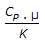a. Grashoff b. Nusselt c. Prandtl d. Stanton
 20. Heat transfer co-efficient (h) for a fluid flowing inside a clean pipe is given by following equation. This is valid for the value of NRe equal to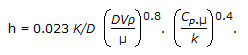a. < 2100 b. 2100-4000 c. > 4000 d. > 10000